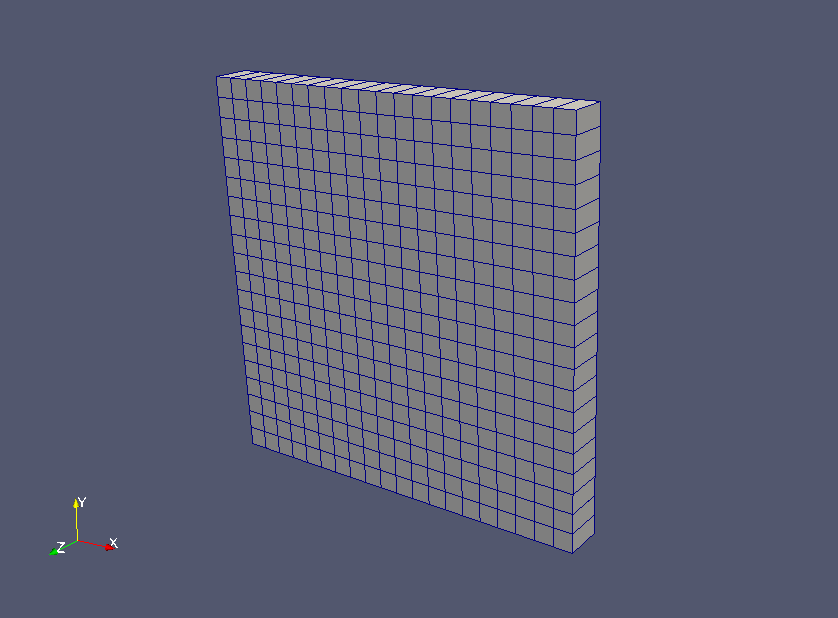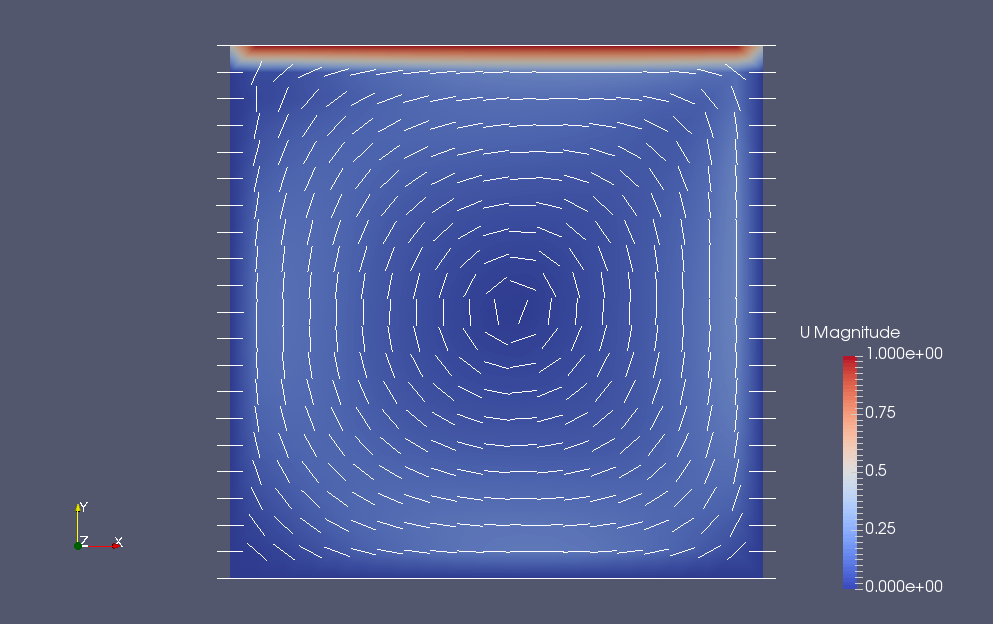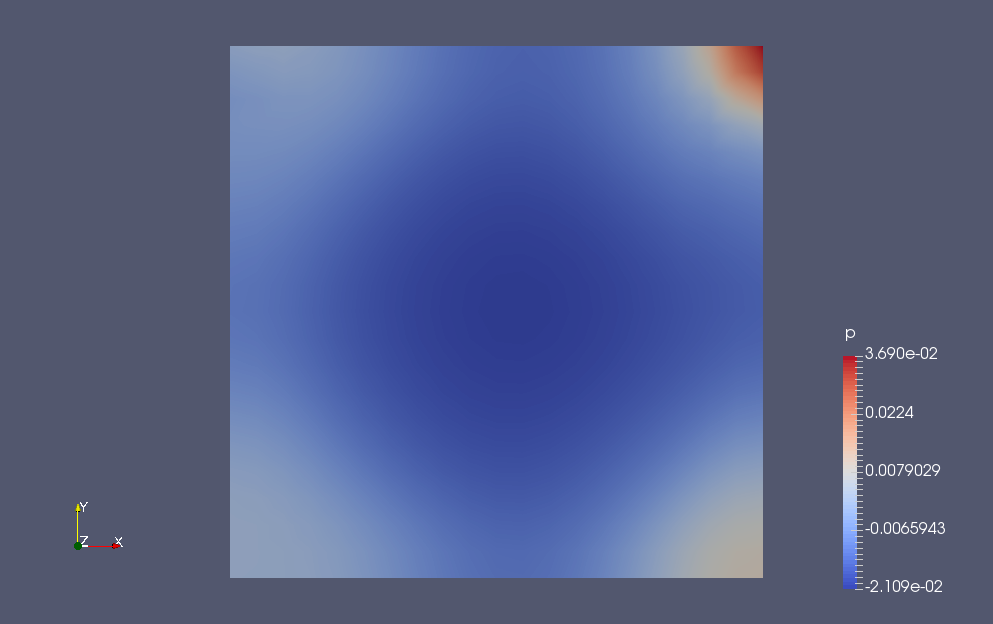﻿ Cavity flow (PBiCCCG solver) - XSim

# Cavity flow (PBiCCCG solver)

Update: June 1, 2017
OpenFOAM 4.x

## Case directory

\$FOAM_TUTORIALS/incompressible/pisoFoam/ras/cavityCoupledU

## Summary

We calculate the flow of a system where the upper wall "movingWall" is moving in parallel at 1 m/s and the other walls "fixedWalls" are stationary from 0 sec to 10 sec. There is no inflow or outflow of fluid, and the calculation is performed as a two-dimensional problem with a single mesh in the Z-direction.Model geometry

The standard k-ε model is used for the turbulence model.

For the flow velocity (U) solver, we will use the conjugate type solver PBiCCCG. This setting is specified in the file system/fvSolution as follows.

```U
{
type            coupled;
solver          PBiCCCG;
preconditioner  DILU;
tolerance       (1e-05 1e-05 1e-05);
relTol          (0 0 0);
}
```

The meshes are as follows.Meshes

The calculation result is as follows.

Flow velocity (U)Flow velocity at final time (U)Pressure at final time (p)

We can see that a vortex is being generated.

## Commands

cp -r \$FOAM_TUTORIALS/incompressible/pisoFoam/ras/cavityCoupledU cavityCoupledU
cd cavityCoupledU

blockMesh
pisoFoam

paraFoam

## Calculation time

6.05 seconds *Single, Inter(R) Core(TM) i7-2600 CPU @ 3.40GHz 3.40GHz